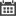## Blog

### Comparing different methods to get the 50th Fibonacci number in C++When comparing different algorithms, we typically look at space and time complexity, and make a trade off decision based on the applications needs. Let’s look at the problem, “find the 50th Fibonacci number,” and see how you [..]

### Introduction to PID Control LoopsA Proportional Integral Derivative controller (PID Controller) is a closed loop control system that is commonly used in industrial control systems. This method is very effective when dealing with an application that requires [..]